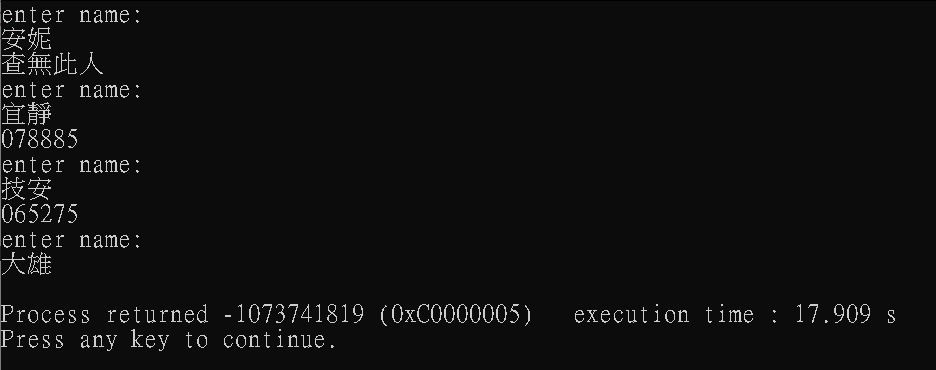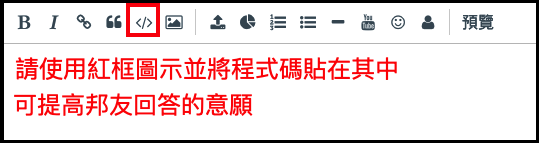#0

## 陣列收尋問題 [使用C語言]

``````#include <stdio.h>
#include <stdlib.h>
#include <string.h>

void main(void) {
char *a = {"大雄","宜靜","技安"};
char *b = {"093656","078885","065275"};
char n[] = "";
int i;

while(1) {
puts("enter name:");
scanf ("%s", &n);
for (i = 0; i < 3; i++) {
if(strcmp(n,a[i]) == 0) {printf ("%s\n", b[i]); break;}
}
if (i == 3) printf ("查無此人\n");
}
}
```````if(strcmp(n,a[i]) == 0) {printf ("%s\n", b[i]);}`

``````char *a = {"大雄","宜靜","技安"};
int b = {"93656","78885","65275"};
``````

→但大雄出來的結果還是怪怪的，不是93656是11515498，且b的初始值怎麼改都不會變

``````char *a = {"","大雄","宜靜","技安"};
char *b = {"","093656","078885","065275"};
``````

→但這樣本質問題依然沒有解決

``````char *a = {"q","大雄","宜靜","技安"};
char *b = {"0900","093656","078885","065275"};
``````

==回覆.海綿寶寶==

``````char n[] = "";
``````

### 1 個回答

1``````#include <stdio.h>
#include <stdlib.h>
#include <string.h>

#define MAX_LENGTH 100

void main(void) {
char a[MAX_LENGTH] = {"大雄","宜靜","技安"};
char b[MAX_LENGTH] = {"093656","078885","065275"};
char n[MAX_LENGTH] = "";
int i;

while(1) {
puts("enter name:");
scanf ("%s", &n);
for (i = 0; i < 3; i++) {
if(strcmp(n,a[i]) == 0) {printf ("%s\n", b[i]); break;}
}
if (i == 3) printf ("查無此人\n");
}
}
``````

`char n[]="";`的寫法

spider iT邦新手 5 級 ‧ 2021-06-21 12:43:29 檢舉